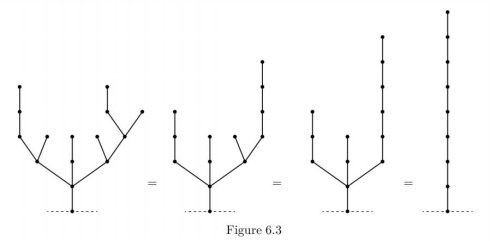# BZOJ2688: Green Hackenbush2018-01-18 题解

## 题解

#### Step2:##### 感性证明

G1、G2拥有相同的SG值意味着两个游戏的总SG值为0，G1+G2的和是一个P局面，也就是必败

$$h[x] = \sum_{i = 0}^{n - 1}{h[i] * h[n - i - 1]}$$

$$g[n][(x + 1) ^ (y + 1)] = \sum_{i = 0}^{n - 1}{ h[i] * g[i][x] * h[n - 1 - i] * g[n - 1 - i][y]}$$

f[i][j]表示的是前i颗子树异或值为j的概率

$$f[i][j ^ k]=f[i-1][j] * g[a[i]][k]$$

$ans = 1-f[n]$